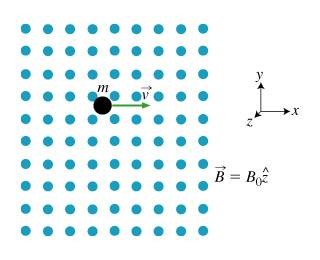# Charge moving in cyclotron orbit

## Homework Statement

The particle moves in a plane perpendicular to the magnetic field direction as shown in the figure. What is omega, the angular frequency of the circular motion?

Express omega in terms of q, m, and B_0.

## Homework Equationsomega=2pi/T

## The Attempt at a Solution

I found that At a given moment the particle is moving in the +x direction (and the magnetic field is always in the +z direction). If q is positive, the direction of the force on the particle due to the magnetic field is -y direction

The force on a charged particle in a Magnetic Field B is given by F = q(v X B).

The force required to accelerate a particle of velocity v in a circular motion is related to the radius of the circular path and the mass of the particle by F=(m*v^2)/r

Omega can also be expressed as v/r.

By equating the two forces and playing around a bit i'm sure you'll come out with your answer.

can anyone give the Lagrange of the system in plane polar coordinates?